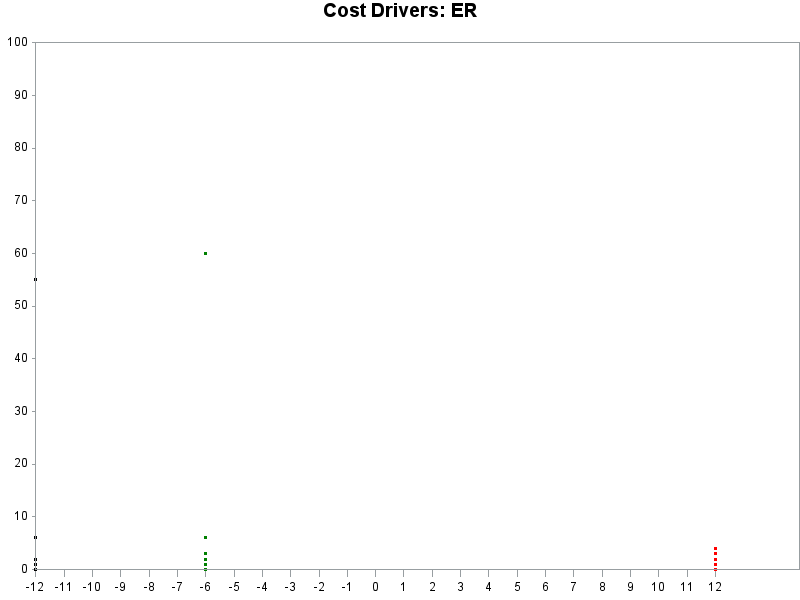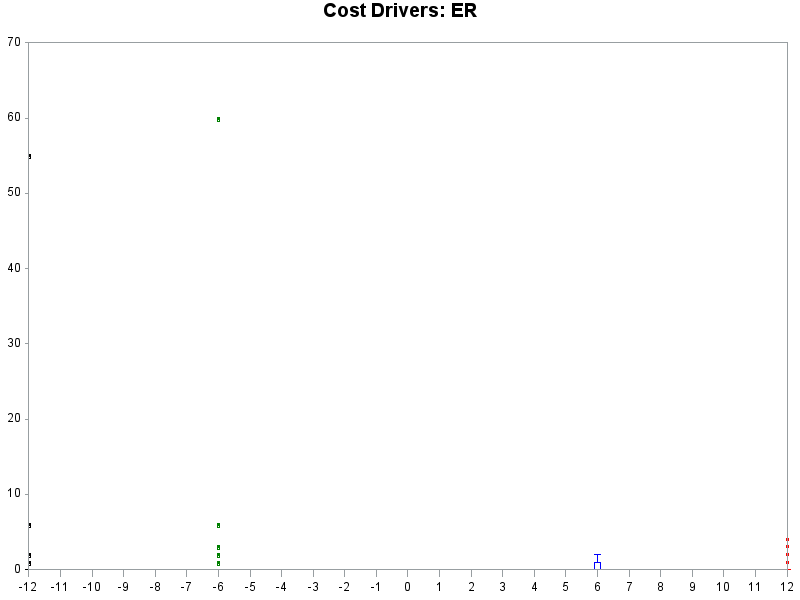## Help creating graph with multiple series

Hello,

I am looking to create a graph with the following characteristics:

• X-Axis: Timepoint (months) -12, -6, 0, 6, 12   *Note: the - refers to that many months before study entry
• Y-Axis: Days in Hospital at 12 months before study entry (coincides with -12 m timepoint)
• Y-Axis: Days in Hospital at 6 months before study entry (coincides with -6 m timepoint)
• Y-Axis: Days in Hospital at 6 months post study entry (coincides with 6 m timepoint)
• Days in Hospital at 12 months post study entry (coincides with 12 m timepoint)

Each of those y-axis variables is one variable in the dataset. I want to create a scatterplot in a series format and then have the mean display on the graph. I tried using ODS graphics designer and am only able to create a scatter for one y-axis variable. Is it possible to do a series for this with the graphics editor? If not, how could I go about doing this?

Thanks!

15 REPLIES 15

## Re: Help creating graph with multiple series

With SAS/Graph Proc Gplot, you can plot multiple different y-variables on the same graph using the 'overlay' option:

If you have 2 different ranges of values, you can use gplot's "plot2" for the 2nd axis (on the right-hand-side):

And if you need more than 2 different y-axes, you could get a little tricky with annotate:

For the mean line, you could calculate that value and store it in a macro variable, and then use that value as a 'refline' or annotate a line at that value.

Are any of these similar to what you're wanting?  (If not, give some more specifics/details of what you're looking for.)

## Re: Help creating graph with multiple series

Thanks for the info... the range of values on the y-axis is the same for all, so won't be necessary to use 2nd axis I don't think.

How would I set this up in Proc Gplot?

## Re: Help creating graph with multiple series

Using some of the easily-available sample data we ship with SAS, here's a simple example:

symbol1 value=dot interpol=join color=red;
symbol2 value=dot interpol=join color=blue;
symbol3 value=dot interpol=join color=green;

proc gplot data=sashelp.class;
plot age*name=1 weight*name=2 height*name=3 / overlay;
run;

## Re: Help creating graph with multiple series

Thanks, that works. How do I get the plot to not join lines between the datapoints? I just want to show the datapoints on the graph but do not want the points joined in any way.

## Re: Help creating graph with multiple series

If you're asking about gplot ... you can use interpol=none, rather than interpol=join

## Re: Help creating graph with multiple series

Thanks that works! How do I get the x and y axis to join at 0 so that -12 and -6 month timepoints are to the left of the y axis?

## Re: Help creating graph with multiple series

You can specify axis statements (with whatever range you want, using the order= axis option), and assign them to the graph using the haxis and vaxis gplot options.

## Re: Help creating graph with multiple series

I've attached the plot that I have right now and my code is at the bottom of this question screen. Here's what I still need to do with it/some questions:

• Add means as bars on the graph to designate the average of each of the four variables (ERDAYS_PRE_3 ERDAYS_PRE_4 ERDAYS_3 ERDAYS_4)
• Move the y-axis so that it is at 0
• Add in values for ERDAYS_3 - for some reason they don't show up on the graph?

CODE:

/*Setup plot for ER cost driver data*/

symbol1 value=dot interpol=none color=red;

symbol2 value=dot interpol=none color=blue;

symbol3 value=dot interpol=none color=green;

symbol4 value=dot interpol=none color=black;

axis1 order=(-12 to 12 by 1) offset=(0,12)

label=none minor=none;

axis2 order=(0 to 100 by 10) offset=(0,0)

label=none minor=none;

proc gplot data=CostDrivers;

plot ERDAYS_PRE_3*time_6=3 ERDAYS_PRE_4*time_12=4 ERDAYS_4*time12=1 ERDAYS_3*time6=2  / overlay

haxis=axis1

vaxis=axis2;

run;

Thanks for your help!## Re: Help creating graph with multiple series

When you say "add means as bars" - how about a boxplot?

(you can use interpol=boxcti in your symbol statement, for example)

Not quite sure what you're wanting when you say "move the y axis so that it is at zero".   Your y axis is starting at zero (as per your axis statement), but I'm not sure how you're wanting to adjust it(?)

If the ERDAYS_3 aren't showing up in the graph, it's likely that their values aren't in the range specified in the axis statemen(?)  Try doing a proc print and look at the values.  Or alternatively temporarily comment out the axis options and see where/how the values plot, and see if they are outside the desired range.

## Re: Help creating graph with multiple series

Yes a boxplot would be great. When I updated it that way the only values that show up with the boxplot are for timepoint 6. For the y-axis, I want it to be at x=0 so that the timepoints -12 and -6 are to the left of the y axis.

Code:

symbol1 interpol=boxcti color=red;

symbol2 interpol=boxcti color=blue;

symbol3 interpol=boxcti color=green;

symbol4 interpol=boxcti color=black;

axis1 order=(-12 to 12 by 1) offset=(0,0)

label=none minor=none;

axis2 order=(0 to 70 by 10) offset=(0,0)

label=none minor=none;

proc gplot data=CostDrivers;

plot ERDAYS_PRE_3*time_6=3 ERDAYS_PRE_4*time_12=4 ERDAYS_4*time12=1 ERDAYS_3*time6=2  / overlay

haxis=axis1

vaxis=axis2;

run;

quit;

Here's the updated graph:## Re: Help creating graph with multiple series

To get both the boxplot, and the points, to show up, you have to plot them both.  Something like this...

symbol1 interpol=boxcti color=red;

symbol2 interpol=boxcti color=blue;

symbol3 interpol=boxcti color=green;

symbol4 interpol=boxcti color=black;

symbol5 value=dot interpol=none color=red;

symbol6 value=dot interpol=none color=blue;

symbol7 value=dot interpol=none color=green;

symbol8 value=dot interpol=none color=black;

plot

ERDAYS_PRE_3*time_6=3 ERDAYS_PRE_4*time_12=4 ERDAYS_4*time12=1 ERDAYS_3*time6=2

ERDAYS_PRE_3*time_6=7 ERDAYS_PRE_4*time_12=8 ERDAYS_4*time12=5 ERDAYS_3*time6=6

/ overlay

The y-axis will always show up at the far-left of the graph.  There's no option to have it show up in the middle.  You could possibly draw an axis in the middle with annotate, but that would take a bit of work...

## Re: Help creating graph with multiple series

Here's an example where I "fake" the y-axis (using annotate) so I can have it in the middle of the graph:Jay54
Meteorite | Level 14

## Re: Help creating graph with multiple series

Use a Scatter plot instead of Series.  Add reference lines at x=0 and y=0 and turn off the axis lines.  The tick values will still be displayed on the outer edges.

## Re: Help creating graph with multiple series

I think you could also try PROC SGPLOT with either a series or scatter statement.

proc sgplot data=have;

series x=time y=time6;

series x=time y=time12;

series x=time y=time_6;

run;quit;

But from your data it doesn't look like you have 5 series, it looks like you have 5 points really.  Where does the 'series' part come in?

Discussion stats
• 15 replies
• 1391 views
• 0 likes
• 4 in conversation